• +91 9971497814
• info@interviewmaterial.com

# RD Chapter 10- Circles Ex10.2 Interview Questions Answers

### Related Subjects

Question 1 : If PT is a tangent at T to a circle whose centre is O and OP = 17 cm, OT = 8 cm. Find the length of the tangent segment PT.

PT is the tangent to the circle with centre O, at T
Radius OT = 8 cm, OP = 17 cm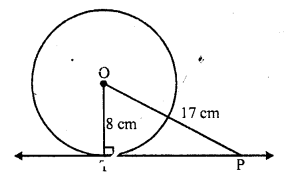PT is the tangent segment
Now in right ∆OPT,
OP² = OT² + PT² (Pythagoras Theorem)
=> (17)² = (8)² + PT²
=> 289 = 64 + PT²
=> PT² = 289 – 64 = 225 = (15)²
PT = 15 cm

Question 2 : Find the length of a tangent drawn to a circle with radius 5 cm, from a point 13 cm from the centre of the circle.

From a point P outside the circle with centre O, PT is the tangent to the circle and radius
OT = 5 cm, OP = 15 cm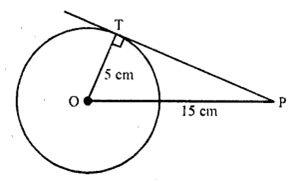OT ⊥ PT
Now in right ∆OPT,
OP² = OT² + PT² (Pythagoras Theorem)
(13)² = (5)² + PT²
=> 169 = 25 + PT²
=> PT² = 169 – 25 = 144 = (12)²
PT = 12 cm

Question 3 : A point P is 26 cm away from the centre O of a circle and the length PT of the tangent drawn from P to the circle is 10 cm. Find the radius of the circle.

From a point P outside the circle of centre 0 and radius OT, PT is the tangent to the circle
OP = 26 cm, PT = 10 cm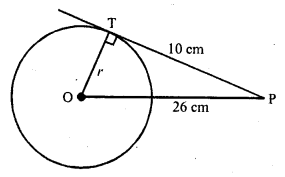Now in right ∆OPT
OP² = OT² + PT² (Pythagoras Theorem)
=> (26)² = r² + (10)²
=> 676 = r² + 100
=> 676 – 100 = r²
=> r² = 576 = (24)²
r = 24
Hence radius of the circle = 24 cm

Question 4 : If from any point on the common chord of two intersecting circles, tangents be drawn to the circles, prove that they are equal.

Given : QR is the common chord of two circles intersecting each other at Q and R
P is a point on RQ when produced From PT and RS are the tangents drawn to tire circles with centres O and C respectively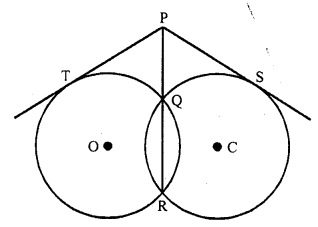To prove : PT = PS
Proof: PT is the tangent and PQR is the secant to the circle with centre O
PT² = PQ x PR ….(i)
Similarly PS is the tangent and PQR is the secant to the circle with centre C
PS² = PQ x PR ….(ii)
From (i) and (ii)
PT² = PS²
PT = PS
Hence proved.

Question 5 : If the sides of a quadrilateral touch a circle, prove that the sum of a pair of opposite sides is equal to the sum of the other pair.

Given : The sides of a quadrilateral ABCD touch the circle at P, Q, R and S respectively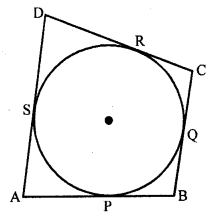To prove : AB + CD = AP + BC
Proof : AP and AS are the tangents to the circle from A
AP = AS ….(i)
Similarly BP = BQ ……(ii)
CR = CQ ….(iii)
and DR = DS ….(iv)
AP + BP + CR + DR = AS + BQ + CQ + DS
=> (AP + BP) + (CR + DR) = (AS + DS) + (BQ + CQ)
=> AB + CD = AD + BC
Hence proved.

Question 6 : Out of the two concentric circles, the radius of the outer circle is 5 cm and the chord AC of length 8 cm is a tangent to the inner circle. Find the radius of the inner circle. [NCERT Exemplar]

Let C1 and C2 be the two circles having same centre O. AC is a chord which touches the C1 at point D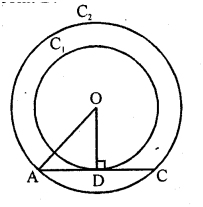Join OD.
Also, OD ⊥ AC
AD = DC = 4 cm
[perpendicular line OD bisects the chord]
In right angled ∆AOD,
[by Pythagoras theorem, i.e.,
(hypotenuse)² = (base)² + (perpendicular)²]
=> DO² = 5² – 4² = 25 – 16 = 9
=> DO = 3 cm
Radius of the inner circle OD = 3 cm

Question 7 : A chord PQ of a circle is parallel to the tangent drawn at a point R of the circle. Prove that R bisects the arc PRQ. [NCERT Exemplar]

Given : Chord PQ is parallel tangent at R.
To prove : R bisects the arc PRQ.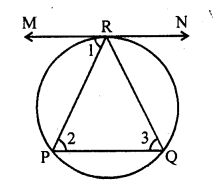Proof: ∠1 = ∠2 [alternate interior angles]
∠1 = ∠3
[angle between tangent and chord is equal to angle made by chord in alternate segment]
∠2 = ∠3
=> PR = QR
[sides opposite to equal angles are equal]
=> PR = QR
So, R bisects PQ.

Question 8 : Prove that a diameter AB of a circle bisects all those chords which are parallel to the tangent at the point A. [NCERT Exemplar]

Given, AB is a diameter of the circle.
A tangent is drawn from point A.
Draw a chord CD parallel to the tangent MAN.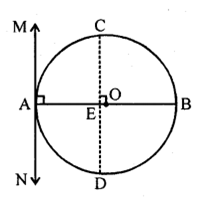So, CD is a chord of the circle and OA is a radius of the circle.
∠MAO = 90°
[Tangent at any point of a circle is perpendicular to the radius through the point of contact]
∠CEO = ∠MAO [corresponding angles]
∠CEO = 90°
Thus, OE bisects CD
[perpendicular from centre of circle to chord bisects the chord]
Similarly, the diameter AB bisects all. Chord which are parallel to the tangent at the point A.

Question 9 : If AB, AC, PQ are the tangents in the figure, and AB = 5 cm, find the perimeter of ∆APQ.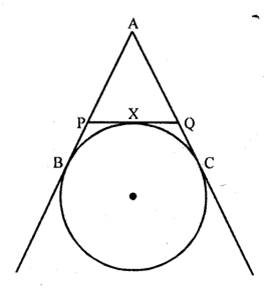Given : AB, AC and PQ are the tangents to the circle as shown in the figure above and AB = 5 cm
To find : The perimeter of ∆APQ
Proof: PB and PX are the tangents to the circle
PB = PX
Similarly QC and QX are the tangents from
QC = QX
and AB and AC are the tangents from A
AB = AC
Now perimeter of ∆APQ
= AP + PQ + AQ
= AP + PX + QX + AQ
= AP + PB + QC + AQ { PB = PX and QC = QX}
= AB + AC
= AB + AB (AB=AC)
= 2 AB = 2 x 5 = 10 cm

Question 10 : Prove that the intercept of a tangent between two parallel tangents to a circle subtends a right angle at the centre.

Given : PQ and RS are parallel tangents of a circle
RMP is the intercept of the tangent between PQ and RS
RO and PQ are joined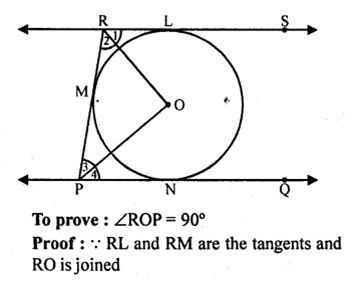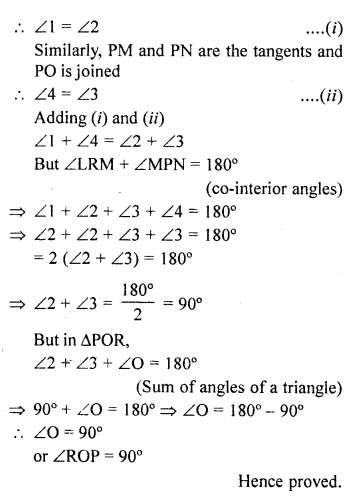Todays Deals### RD Chapter 10- Circles Ex10.2 Contributorskrishan

Name:
Email:

# Latest News# 9000 interview questions in different categories